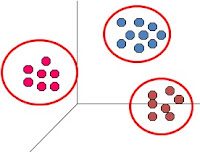## Pages

### Difference between K-Means and Hierarchical Clustering - Usage Optimization### When should I go for K-Means Clustering and when for Hierarchical Clustering ?

Often people get confused, which one of the two i.e. K-Means Clustering, and Hierarchical Clustering, techniques should be used for performing a Cluster Analysis.

Well, Answer is pretty simple, if your data is small then go for Hierarchical Clustering and if it is large then go for K-Means Clustering.

Why ???

Are you really interested in knowing background story ...?

All right !

In Hierarchical Clustering, first all the possible distances among the observations are calculated. With the basic Knowledge of Permutation and Combinations, we know that the number of Distances would be
n
No. of Pairs =           C ,  where n is number of observations.
2

Now once the nearby observation make pair, the distances among newly formed pairs are calculated.

Imagine the number of distances if n = 5, in first iteration, it would be  5! / ( 2! * 3!) = 10, which are manageable.
However if n = 10,000 then number of distances = (10000! / ( 2! * 9998!)
Now (10000! / ( 2! X 9998!)  = 10000 X 9999 / 2 = 49,99,500

And this is only first iteration. Despite in every iteration the number of distance reduce significantly,  calculation of these many distances become quite un-manageable.

Hence we switch to K-Means Clustering.

In K-Means Clustering, Suppose we go for  K = 3 clusters, then all the observation are divided into 3 Clusters in purely random fashion, and 3 Centroids are Calculated

Now Distance of each observation with each Centroid is calculated. So in first iteration, keeping number of observation 10,000 again, the number of distances calculated would be = 3 X 10000 = 30000.

Now again Centroid would be calculated and then again the distances ( 30,000 again).
So even after fair number of iterations, calculation of distances remains quite manageable.

Then one would say, then we should use only K-Means ... well, I would say ... You can.

But in K-Means Clustering,we need to iterate the model to find out the optimal number of Clusters, but in Hierarchical Clustering, it automatically gives result at various number of Clusters.

Time is money, so please make a habit to save it.

Hence, use hierarchical Clustering for small dataset, and K-Means Clustering for large dataset.

Enjoy reading our other articles and stay tuned with ...

Kindly do provide your feedback in the 'Comments' Section and share as much as possible.

1.This comment has been removed by a blog administrator.

2.It is amazing and wonderful to visit your site.Thanks for sharing this information,this is useful to me...
http://chennaitraining.in/base-sas-training-in-chennai/
http://chennaitraining.in/abinitio-training-in-chennai/
http://chennaitraining.in/datastage-training-in-chennai/
http://chennaitraining.in/cognos-training-in-chennai/
http://chennaitraining.in/cognos-tm1-training-in-chennai/
http://chennaitraining.in/microstrategy-training-in-chennai/
http://chennaitraining.in/qlikview-training-in-chennai/

Do provide us your feedback, it would help us serve your better.